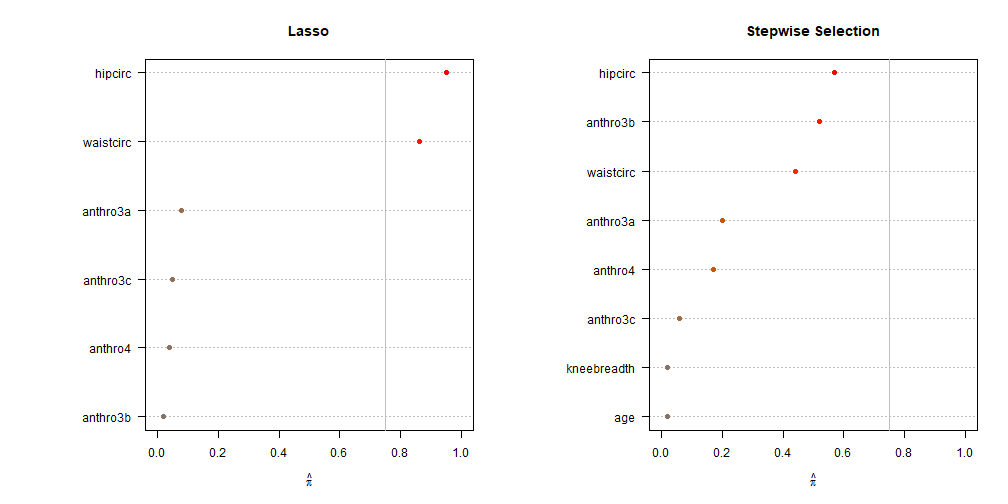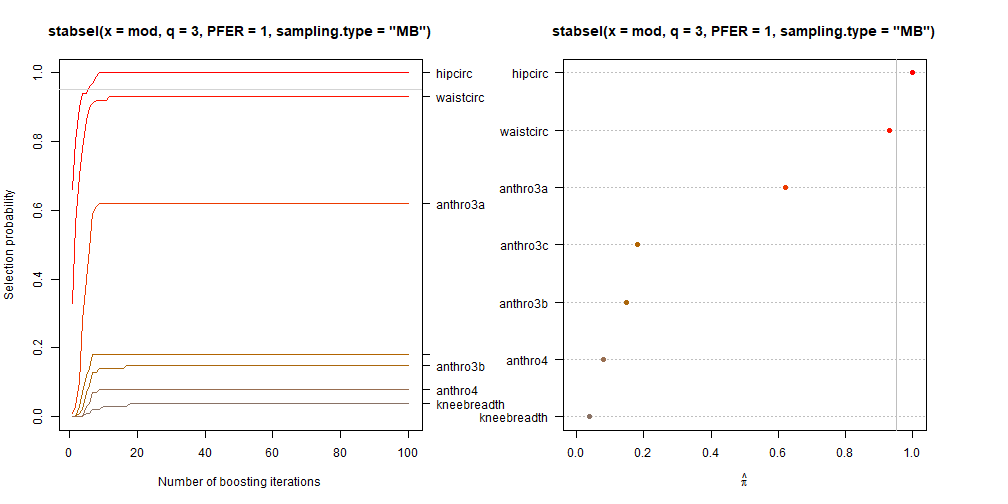stabs implements resampling procedures to assess the stability of selected variables with additional finite sample error control for high-dimensional variable selection procedures such as Lasso or boosting. Both, standard stability selection (Meinshausen & Bühlmann, 2010, doi:10.1111/j.1467-9868.2010.00740.x) and complementarty pairs stability selection with improved error bounds (Shah & Samworth, 2013, doi:10.1111/j.1467-9868.2011.01034.x) are implemented. The package can be combined with arbitrary user specified variable selection approaches.

## Installation

• Current version (from CRAN):
``````install.packages("stabs")
``````
• Latest development version from GitHub:
``````library("devtools")
install_github("hofnerb/stabs")
``````

To be able to use the `install_github()` command, one needs to install devtools first:

``````install.packages("devtools")
``````

## Using stabs

A simple example of how to use stabs with package lars:

``````library("stabs")
``````
``````## Loading required package: parallel
``````
``````library("lars")
``````
``````## Loaded lars 1.2
``````
``````## make data set available
data("bodyfat", package = "TH.data")
## set seed
set.seed(1234)

## lasso
(stab.lasso <- stabsel(x = bodyfat[, -2], y = bodyfat[,2],
fitfun = lars.lasso, cutoff = 0.75,
PFER = 1))
``````
``````##  Stability Selection with unimodality assumption
##
## Selected variables:
## waistcirc   hipcirc
##         2         3
##
## Selection probabilities:
##         0.00         0.00         0.00         0.02         0.04         0.05
##     anthro3a    waistcirc      hipcirc
##         0.08         0.86         0.95
##
## ---
## Cutoff: 0.75; q: 2; PFER (*):  0.454
##    (*) or expected number of low selection probability variables
## PFER (specified upper bound):  1
## PFER corresponds to signif. level 0.0504 (without multiplicity adjustment)
``````
``````## stepwise selection
(stab.stepwise <- stabsel(x = bodyfat[, -2], y = bodyfat[,2],
fitfun = lars.stepwise, cutoff = 0.75,
PFER = 1))
``````
``````##  Stability Selection with unimodality assumption
##
## No variables selected
##
## Selection probabilities:
##         0.00         0.02         0.02         0.06         0.17         0.20
##    waistcirc     anthro3b      hipcirc
##         0.44         0.52         0.57
##
## ---
## Cutoff: 0.75; q: 2; PFER (*):  0.454
##    (*) or expected number of low selection probability variables
## PFER (specified upper bound):  1
## PFER corresponds to signif. level 0.0504 (without multiplicity adjustment)
``````

Now plot the results

``````## plot results
par(mfrow = c(1, 2))
plot(stab.lasso, main = "Lasso")
plot(stab.stepwise, main = "Stepwise Selection")
``````We can see that stepwise selection seems to be quite unstable, even in this low dimensional example!

### User-specified variable selection approaches

To use stabs with user specified functions, one can specify an own `fitfun`. These need to take arguments `x` (the predictors), `y` (the outcome) and `q` the number of selected variables as defined for stability selection. Additional arguments to the variable selection method can be handled by `...`. In the function `stabsel()` these can then be specified as a named list which is given to `args.fitfun`.

The `fitfun` function then needs to return a named list with two elements `selected` and `path`:

• `selected` is a vector that indicates which variable was selected.
• `path` is a matrix that indicates which variable was selected in which step. Each row represents one variable, the columns represent the steps. The latter is optional and only needed to draw the complete selection paths.

The following example shows how `lars.lasso` is implemented:

``````lars.lasso
``````
``````## function (x, y, q, ...)
## {
##     if (!requireNamespace("lars", quietly = TRUE))
##         stop("Package ", sQuote("lars"), " needed but not available")
##     if (is.data.frame(x)) {
##         message("Note: ", sQuote("x"), " is coerced to a model matrix without intercept")
##         x <- model.matrix(~. - 1, x)
##     }
##     fit <- lars::lars(x, y, max.steps = q, ...)
##     selected <- unlist(fit\$actions)
##     if (any(selected < 0)) {
##         idx <- which(selected < 0)
##         idx <- c(idx, which(selected %in% abs(selected[idx])))
##         selected <- selected[-idx]
##     }
##     ret <- logical(ncol(x))
##     ret[selected] <- TRUE
##     names(ret) <- colnames(x)
##     cf <- fit\$beta
##     sequence <- t(cf != 0)
##     return(list(selected = ret, path = sequence))
## }
## <bytecode: 0x0000000024b2bef8>
## <environment: namespace:stabs>
``````

To see more examples simply print, e.g., `lars.stepwise`, `glmnet.lasso`, or `glmnet.lasso_maxCoef`. Please contact me if you need help to integrate your method of choice.

### Using boosting with stability selection

Instead of specifying a fitting function, one can also use `stabsel` directly on computed boosting models from mboost.

``````library("stabs")
library("mboost")
### low-dimensional example
mod <- glmboost(DEXfat ~ ., data = bodyfat)

## compute cutoff ahead of running stabsel to see if it is a sensible
## parameter choice.
##   p = ncol(bodyfat) - 1 (= Outcome) + 1 ( = Intercept)
stabsel_parameters(q = 3, PFER = 1, p = ncol(bodyfat) - 1 + 1,
sampling.type = "MB")
``````
``````## Stability selection without further assumptions
##
## Cutoff: 0.95; q: 3; PFER:  1
## PFER (specified upper bound):  1
## PFER corresponds to signif. level 0.1 (without multiplicity adjustment)
``````
``````## the same:
stabsel(mod, q = 3, PFER = 1, sampling.type = "MB", eval = FALSE)
``````
``````## Stability selection without further assumptions
##
## Cutoff: 0.95; q: 3; PFER:  1
## PFER (specified upper bound):  1
## PFER corresponds to signif. level 0.1 (without multiplicity adjustment)
``````
``````## now run stability selection
(sbody <- stabsel(mod, q = 3, PFER = 1, sampling.type = "MB"))
``````
``````##  Stability Selection without further assumptions
##
## Selected variables:
## hipcirc
##       4
##
## Selection probabilities:
##         0.00         0.00         0.00         0.04         0.08         0.15
##     anthro3c     anthro3a    waistcirc      hipcirc
##         0.18         0.62         0.93         1.00
##
## ---
## Cutoff: 0.95; q: 3; PFER:  1
## PFER corresponds to signif. level 0.1 (without multiplicity adjustment)
``````

Now let us plot the results, as paths and as maximum selection frequencies:

``````opar <- par(mai = par("mai") * c(1, 1, 1, 2.7), mfrow = c(1, 2))
plot(sbody, type = "paths")
plot(sbody, type = "maxsel", ymargin = 6)
````````````par(opar)
``````

## Citation

To cite the package in publications please use

``````citation("stabs")
``````

which will currently give you

``````##
## To cite package 'stabs' in publications use:
##
##   Benjamin Hofner and Torsten Hothorn (2021). stabs: Stability
##   Selection with Error Control, R package version 0.6-4,
##   https://CRAN.R-project.org/package=stabs.
##
##   Benjamin Hofner, Luigi Boccuto and Markus Goeker (2015). Controlling
##   false discoveries in high-dimensional situations: Boosting with
##   stability selection. BMC Bioinformatics, 16:144.
##   doi:10.1186/s12859-015-0575-3
##
## To cite the stability selection for 'gamboostLSS' models use:
##
##   Thomas, J., Mayr, A., Bischl, B., Schmid, M., Smith, A., and Hofner,
##   B. (2017). Gradient boosting for distributional regression - faster
##   tuning and improved variable selection via noncyclical updates.
##   Statistics and Computing. Online First. DOI 10.1007/s11222-017-9754-6
##
## Use 'toBibtex(citation("stabs"))' to extract BibTeX references.
## To see these entries in BibTeX format, use 'print(<citation>,
## bibtex=TRUE)', 'toBibtex(.)', or set
## 'options(citation.bibtex.max=999)'.
``````

To obtain BibTeX references use

``````toBibtex(citation("stabs"))
``````